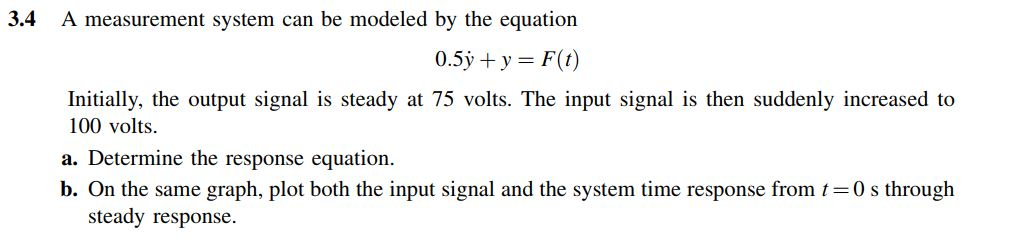# Question Solved1 Answer3.4 A measurement system can be modeled by the equation 0.5y + y = F(t) Initially, the output signal is steady at 75 volts. The input signal is then suddenly increased to 100 volts. a. Determine the response equation. b. On the same graph, plot both the input signal and the system time response from t=0 s through steady response.X8PQNF The Asker · Mechanical EngineeringTranscribed Image Text: 3.4 A measurement system can be modeled by the equation 0.5y + y = F(t) Initially, the output signal is steady at 75 volts. The input signal is then suddenly increased to 100 volts. a. Determine the response equation. b. On the same graph, plot both the input signal and the system time response from t=0 s through steady response.
More
Transcribed Image Text: 3.4 A measurement system can be modeled by the equation 0.5y + y = F(t) Initially, the output signal is steady at 75 volts. The input signal is then suddenly increased to 100 volts. a. Determine the response equation. b. On the same graph, plot both the input signal and the system time response from t=0 s through steady response.